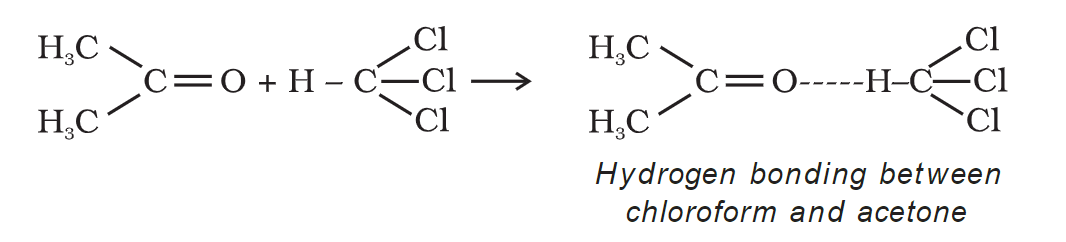Checkout JEE MAINS 2022 Question Paper Analysis : Checkout JEE MAINS 2022 Question Paper Analysis :

# Chemistry Practical Class 12 Determination of enthalpy change during interaction (Hydrogen bond formation) between acetone and chloroform Viva Questions with Answers

Q1. Chloroform and acetone do not form an ideal liquid pair, whereas acetone and benzene do form. Why?

Answer. In a mixture of chloroform and acetone, there will be hydrogen bonding. Therefore, there will be a negative deviation from Raoult’s law and therefore, they do not form an ideal liquid pair. In acetone and benzene, there is no such phenomenon taking place.

Q2. Why does the liquid pair of ethanol and water show a positive deviation from Raoult’s law?

Answer. A solution of ethyl alcohol and water deviates from Raoult’s law in a positive way because there is hydrogen bonding in the ethyl alcohol solution and water molecules tend to occupy the space between them, causing some hydrogen bonds to break.

Q3. Give two examples of each of the liquid pairs for which ΔMixing H is negative and positive respectively.

Answer. Two examples of the liquids pairs for which ∆mixing H is negative are:-

• Chloroform + Benzene
• Acetone + Aniline

Two examples of the liquids pairs for which ∆mixing H is positive are:-

• Acetone + Benzene
• Methyl alcohol + water

Q4. How is the vapour pressure of the liquids related to the interaction pattern between the molecules of the components of a liquid mixture?

Answer. In comparison to a liquid’s solution, a pure liquid has a higher vapour pressure. It is inversely proportional to the forces of attraction that exist between liquid molecules. It increases as the temperature rises.

Q5. What is the primary interaction between acetone and chloroform?

Answer. Since each molecule contains a net dipole moment, acetone and chloroform are both polar molecules. As a result, the dipole-dipole force is the dominant force between acetone and chloroform.

Q6. What type of deviation does a mixture of chloroform and acetone exhibit, and why?

Answer. A solution of chloroform and acetone deviates from Raoult’s law because they have an attractive interaction, which results in the formation of hydrogen bonding. As a result, they deviate from Raoult’s law in a negative way.

Q7. What happens if you mix acetone and chloroform?

Answer. When acetone and chloroform are mixed, hydrogen bonds form between them. Acetone and chloroform will form a minimum boiling azeotrope at a specific composition.

Q8. Explain why acetone and chloroform deviate from Raoult’s law?

Answer. Acetone and chloroform form a non-ideal liquid pair system that deviates from Raoult’s law. This negative deviation from Raoult’s law implies that when the two components mix, they are strongly held together in liquid form due to hydrogen bonding. In the pure state, however, only weak Van der Waal’s forces hold chloroform and acetone molecules together. The hydrogen bonding between acetone and chloroform molecules is depicted as follows:Q9. Define Raoult Law.

Answer. The mole fraction of any solvent is directly proportional to the vapour pressure of an ideal solution, according to this law. It means that when two liquid pairs interact, they behave optimally.

Q10. What is the formula of acetone?

Answer. The formula of acetone is C3H6O.

Q11. What is the formula of chloroform?

Answer. The formula of chloroform is CHCl3.

Q12. Why does enthalpy change occurs in this process?

Answer. Enthalpy change occurs in this process as a result of hydrogen bond formation.

Q13. Why is the heat change reported for a specific amount?

Answer. Since enthalpy change is a broad thermodynamic property, the heat emitted by the system is proportional to the amount of liquid components mixed. As a result, the heat change is reported for a specified amount. As a result, the enthalpy change during the mixing of 1 mol chloroform and 1 mol acetone is observed.

Q14. How can you correlate the heat evolved from the system with the strength of the hydrogen bond?

Answer. The stronger the Hydrogen bond, the more will be the heat evolved from the system.

Q15. What is hydrogen bonding?

Answer. Hydrogen bonding is the formation of hydrogen bonds, which are a type of attractive intermolecular force caused by the dipole-dipole interaction between a hydrogen atom bonded to a highly electronegative atom and another highly electronegative atom in the vicinity of the hydrogen atom.

Q16. What are the precautions that should be taken while performing the experiment?

Answer. Precautions that should be taken while performing th experiment are as follows:

1. Carefully measure the chloroform and acetone.
2. With a thermometer calibrated to 0.1°C, carefully record the temperature.

Q17. What is the Importance of Enthalpy Change?

Answer. The enthalpy change that occurs during the interaction of acetone and chloroform is important because-

• It provides a new path to finding the temperature rise.
• It aids in determining whether the reaction is endothermic or exothermic.
• It also aids in the throttling or Joule-Thomson expansion process.
• It is used to calculate heat flow and heat reactions.
• It also measures the compressor’s power.

Download Class 12 Chemistry Viva questions on the Determination of enthalpy change during interaction (Hydrogen bond formation) between acetone and chloroform by clicking on the button below.